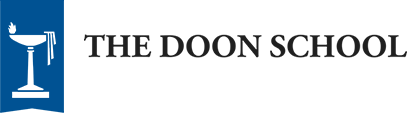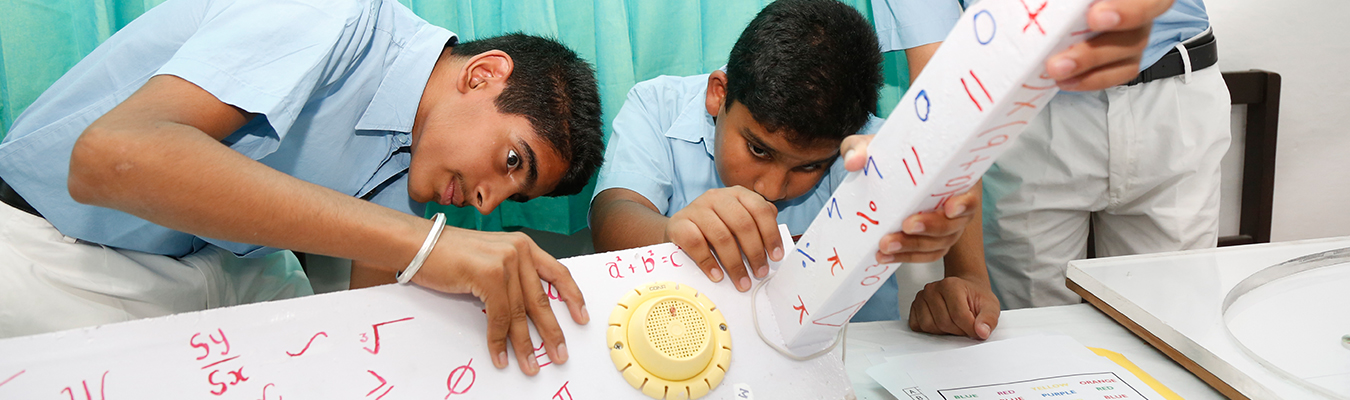### Mathematics

### News Categories

Department:Department of Mathematics

Subject:Mathematics

#### Course Content

D Form

• Pure Arithmetic: Number System, Factors and Multiples, Fractions, Decimals, Powers and Roots
• Commercial Arithmetic: Unitary Method, Distance, Time and Speed, Ratio and Proportion, Percentage, Profit, Loss and Discount, Simple Interest, Average
• Algebra: Fundamental Concepts and Operations, Special Products, Factorisation, Simplification of Algebraic Fractions, Formula, Exponents, Linear Equations in One Variable, Graphs of linear equations
• Geometry: Basic Geometric Concepts, Parallel Lines, Triangles, Polygons, Basic Constructions
• Mensuration: Perimeter and area of rectangles and squares, Volume and Surface Area of cubes and cuboids
• Statistics: Graphical Representation of Data, Frequency Distribution, Mean

C Form

• Sets: Roster and Set Builder forms, Types of sets, Operations on sets, Venn Diagrams
• Pure Arithmetic: Number System, Factors and Multiples, Fractions, Decimals, Squares and Square Roots, Cubes and Cube Roots
• Commercial Arithmetic: Unitary Method, Ratio and Proportion, Distance, Time and Speed, Percentage, Profit, Loss and Discount, Simple and Compound Interest
• Algebra: Fundamental Concepts and Operations, Formula, Exponents, Special Products, Factorisation, Simplification of Algebraic Expressions, Linear Equations in One Variable, Simultaneous Linear Equations, Quadratic Equations, Graphs of linear equations
• Geometry: Basic Geometric Concepts, Triangles, Polygons, Circle, Constructions
• Mensuration: Perimeter and area of triangles and quadrilaterals, Circumference and Area of Circle, Volume and Surface Area of cubes and cuboids
• Statistics: Graphical Representation of Data, Frequency Distribution, Mean, Median and Mode

#### Learning Objectives

• To give students an understanding of fundamental concepts of Mathematics suitable to their age group
• To build inductive and deductive reasoning of students
• To bring awareness in students about role of Mathematics in everyday life thus building the awareness of applications of Mathematics in various other fields
• To prepare students for ICSE curriculum of B and A forms

#### Skills Acquired by Students

• Knowledge of basic concepts of Mathematics
• Performing simple calculations correctly and learning of basic formulas of Arithmetic, Algebra and Geometry
• Understanding of simple applications of the above-mentioned topics in daily life

Mathematics is a subject in which children take a lot of interest from the primary classes and with growing demand of innovations in teaching techniques worldwide, new ways of teaching Mathematical concepts are implemented to avoid restricting Mathematics to a rote learning subject.

The Mathematics syllabus of C and D forms is designed and taught in such a way that

• It strengthens the understanding of concepts already studied by the students up to Grade 6.
• It develops the interest of students in studying Mathematics as a subject and motivates them to think independently, approach any problem with a logical reasoning, frame a method to simplify the problem, obtain a desired result, analyze and cross-check the result obtained.
• It enables students to correlate their knowledge of different topics and thus come to the conclusion that they have to combine the understanding of these topics to solve a high order problem.
• It enables students to understand the relation between Mathematics and its importance in the modern world.
• It also creates a fun-loving approach to the subject and encourages students to pursue it at higher level.

Department:Department of Mathematics

Subject:Mathematics

Curriculum::ICSE

#### Course Content

B Form

• Pure Arithmetic: Irrational numbers
• Commercial Mathematics: Profit and loss, Compound interest
• Algebra: Expansions, Factorisation, Changing the Subject of a Formula, Linear Equations and Simultaneous Linear Equations, Indices/ Exponents, Logarithms
• Geometry: Triangles, Relation between Sides and Angles of Triangles. Types of Triangles, Congruent Triangles, Constructions (using ruler and compasses), Mid Point Theorem and Its Converse, Equal Intercept Theorem, Similarity, Conditions of Similar Triangles, Pythagoras Theorem, Rectilinear Figures
• Statistics: Introduction, Collection of Data, Presentation of Data, Graphical Representation of Data, Mean, Median of Ungrouped Data.
• Mensuration: Area and Perimeter of a Triangle and a Quadrilateral; Area and Circumference of a Circle; Surface Area and Volume of Cube, Cuboids and Cylinder
• Trigonometry: Trigonometric Ratios, Trigonometric Ratios of Standard Angles – 0, 30, 45, 60, 90 Degrees, Simple 2-D Problems Involving One Right-Angled Triangle, Concept of Sine and Cosine Being Complementary with Simple, Direct Application
• Co-ordinate Geometry: Cartesian System, Plotting a Point in the Plane for Given Coordinates. Graphs of x=0, y=0, x=a, y=a, x=y, y= mx+c Including Identification and Conceptual Understanding of Slope and y-intercept, Recognition of Graphs Based on the Above
• Internal Assessment: A minimum of three assignments are to be done during the year as prescribed by the teacher.

A Form

• Commercial Arithmetic: Compound Interest, Sales Tax and Value Added Tax, Banking and Shares and Dividends
• Algebra: Linear Inequations, Quadratic Equations. Reflection, Ratio and Proportion, Factorization, Matrices and Co-ordinate Geometry
• Geometry: Symmetry, Similarity, Loci, Circles and Constructions
• Mensuration: Area and Circumference of Circle, Area and Volume of Solids – Cone, Sphere
• Trigonometry: Using Identities to Solve/Prove Simple Algebraic Trigonometric Expressions, Trigonometric Ratios of Complementary Angles and Direct Application, Heights and Distances
• Statistics: Basic Concepts, Histogram and Ogive, Mean , Median and Mode
• Probability
• Internal Assessment: A minimum of three assignments are to be done during the year as prescribed by the teacher.

#### Learning Objectives

• To develop mathematical concepts and their applications
• To develop skills to apply the knowledge of Mathematics to other fields
• To prepare students for further studies in Mathematics

#### Skills Acquired by Students

• Applying formulas and performing calculations correctly
• Correlating the knowledge of various topics to apply them in real-life problems
• Understanding of concepts to study them at higher level

• The syllabus for B and A Forms is designed and taught in such a way that:
• It develops an interest in Mathematics and its applications in various fields of life.
• It reinforces logical reasoning, the concept of step-wise method to approach problems.
• It develops the potential of students, encourages independent thinking and prepares them to approach any problem in a systematic way.
• It motivates the students to work in groups for a common goal and appreciate others` work.
• It builds imagination skills of students and teaches them to visualize the problems in different ways.
• It helps the students to learn the mathematical concepts by understanding the logic behind them
• It develops inductive and deductive approaches to solve various problems

Department: Department of Mathematics

Subject: Mathematics

Curriculum: ISC

#### Course Content

S Form

Section A

• Mathematical Reasoning
• Algebra: Complex Numbers, Quadratic Equations, Finite and Infinite Sequences, Permutations and Combinations, Mathematical Induction, Binomial Theorem and Properties of Binomial Coefficients
• Trigonometry: Angles and Arc Lengths, Trigonometric Functions, Compound and Multiple Angles and Trigonometric Equations
• Calculus: Basic Concepts of Relations and Functions, Differential Calculus and Integral Calculus
• Coordinate Geometry: Points and their Coordinates, The Straight Line and Circles
• Statistics: Measures of central tendency, Standard deviation, Combined mean and standard deviation

Section B

• Vectors
• Coordinate Geometry in 3 Dimensions

Section C

• Statistics
• Average Due Date

SC Form

Section A

• Determinants and Matrices
• Boolean Algebra
• Conics: Parabola, Ellipse and Hyperbola
• Inverse Trigonometric Functions
• Calculus: Differential Calculus and Integral Calculus
• Correlation and Regression
• Probability
• Complex Numbers
• Differential Equations

Section B

• Vectors
• Coordinate Geometry in 3 Dimensions: Lines and Planes
• Probability

Section C

• Discount
• Annuities
• Linear Programming
• Application of Derivatives in Commerce and Economics
• Index Numbers and Moving Averages

Note: Students have a choice to attempt either section B or C.

#### Learning Objectives

• To develop an understanding of the terms, concepts and formulas at Senior Secondary level;
• To give deeper understanding of the topics in Mathematics at this level;
• To develop the potential of correlating knowledge of various topics and apply it in various fields.

#### Skills Acquired by Students

• Development of skills to apply mathematical knowledge to solve real life problems
• Using technology (computers and calculators) to enhance their learning
• Application of multiple strategies to approach various problems

The syllabus of S and SC forms is designed and taught in such a way that:

• It develops an understanding of applications of Mathematics in other areas.
• It develops the scientific approach to visualize, analyse and draw conclusions.
• It motivates the students to investigate and explore various areas in which mathematical concepts can be applied.
• It develops logical reasoning, patience and the ability to persist to solve real life problems.
• It teaches the applications of technology (mathematical softwares and various tools) in Mathematics and combining the knowledge of two fields to find solutions to various problems.
• It develops an appreciation for the role of Mathematics in everyday life.

Department: Department of Mathematics

Subject: Mathematics

Curriculum: IB

#### Course Content

S and SC forms

Mathematical Studies SL:

• Topic 1— Number and algebra
• Topic 2— Descriptive statistics
• Topic 3— Logics, sets and probability
• Topic 4— Statistical applications
• Topic 5— Geometry and trigonometry
• Topic 6— Mathematical models
• Topic 7— Introduction to differential calculus
• Project

Mathematics SL:

• Topic 1— Algebra
• Topic 2— Functions and equations
• Topic 3— Circular functions and trigonometry
• Topic 4— Vectors
• Topic 5— Statistics and probability
• Topic 6— Calculus
• Mathematical exploration

Mathematics HL:

Core

• Topic 1— Algebra
• Topic 2— Functions and equations
• Topic 3— Circular functions and trigonometry
• Topic 4— Vectors
• Topic 5— Statistics and probability
• Topic 6— Calculus

Options (one of the topic from topic no. 7, 8, 9 & 10)

• Topic 7 — Statistics and probability
• Topic 8 — Sets, relations and groups
• Topic 9 — Calculus
• Topic 10 — Discrete mathematics
• Mathematical exploration

Further Mathematics:

• Topic 1— Linear algebra
• Topic 2— Geometry
• Topic 3— Statistics and probability
• Topic 4— Sets, relations and groups
• Topic 5— Calculus
• Topic 6— Discrete mathematics

#### Learning Objectives

• To develop an understanding of the terms, concepts and formulas at Senior Secondary level;
• To give deeper understanding of the topics in Mathematics at this level;
• To develop the potential of correlating knowledge of various topics and apply it in various fields.

#### Skills Acquired by Students

• Development of skills to apply mathematical knowledge to solve real life problems
• Using technology (computers and calculators) to enhance their learning
• Application of multiple strategies to approach various problems